﻿ State-variable Synthesis of Electronically-Controllable Sinusoidal OscillatorsPublications are Open
Access in this journal
Article Versions
Export Article
• Normal Style
• MLA Style
• APA Style
• Chicago Style
Research Article
Open Access Peer-reviewed

### State-variable Synthesis of Electronically-Controllable Sinusoidal Oscillators

Manoj Kumar Jain, V. K. Singh, Raj Senani
American Journal of Electrical and Electronic Engineering. 2020, 8(1), 11-20. DOI: 10.12691/ajeee-8-1-2
Received November 11, 2019; Revised December 16, 2019; Accepted January 03, 2020

### Abstract

This paper presents a state-variable synthesis of a class of electronically-controllable sinusoidal oscillator circuits which employ Multiple output second generation controlled current conveyors (CCCII) as active elements, do not require any external passive resistors and employ only two grounded capacitors (GC). The systematic synthesis yields a class of fourteen new oscillators all of which provide independent electronic controls of both the frequency of oscillation and condition of oscillation through separate external bias currents. All the aforementioned features make the synthesized oscillators attractive from the view point of integrated circuit implementation. The workability of the derived circuits has been confirmed from SPICE simulations and some sample results are included. Based upon their performance, evaluated through simulations, the new circuits have been compared with those previously known as well as among themselves and the best circuits of the derived set have been identified.

### 1. Introduction

Sinusoidal oscillators are needed in a wide range of applications in electronics, communication, instrumentation, measurement, control systems etc. Therefore, sinusoidal oscillators have been extensively investigated and their numerous new types of realisations have been proposed in the past using a variety of active circuit building blocks. The survey of the existing literature shows that a large number of circuits have been proposed to realise voltage-mode, current-mode and mixed-mode sinusoidal oscillator circuits. Moreover, there has been a major emphasis in the earlier literature on the realisation of single-element-controlled oscillators using different active building blocks, such as voltage-mode operational amplifiers (VOA), operational transconductance amplifiers (OTA), different types of current conveyors (CC), current feedback operational amplifiers (CFOA), four terminal floating nullors (FTFN), current followers (CF) and voltage followers (VF), current differencing buffered amplifiers (CDBA), current differencing transconductance amplifiers (CDTA), operational trans-resistance amplifiers (OTRA), differential difference complementary current conveyors (DDCCC), differential difference complementary current feedback amplifiers (DDCCFA), differential difference amplifiers (DDA), differential voltage current feedback amplifiers (DVCFA) etc. for instance, see 1, 2, 3, 4, 5, 6, 7, 8, 9, 10, 11, 12, 13, 14, 15, 16, 17, 18, 19, 20 and the references cited therein.

The sinusoidal oscillators being presented in this paper have been synthesized through a state-variable methodology using the translinear second generation current controlled conveyors (CCCIIs) introduced by Fabre-Saaid-Wiest-Boucheron 21, 22 as the active building blocks.

A CCCII has finite input resistance Rx looking into the X-terminal of the CCCII which is given by Rx=VT/2IB (where VT is the thermal voltage) which is electronically controllable by an external bias current IB. Because of this characteristic, the CCCII has been found to be particularly suitable for the realization of electronically-controllable functional circuits, including oscillators. Consequently, during the past two decades, a number of sinusoidal oscillator circuits based on CCCIIs have been reported in the literature 23, 24, 25, 26, 27, 28, 29, 30, 31, 32, 33, 34, 35, 36, 37, 38, 39, 40. However, most of the existing CCCII-based oscillators suffer from one or more of the following drawbacks: (i) employment of dissimilar types of building blocks as in 23, 29, 34, (ii) use of an excessive number of active elements as in 34, 36, 40 (iii) non-availability of independent electronic controls of the frequency of oscillation (FO) and condition of oscillation (CO) both as in 23, 28, 29, 30, 31, 32, 33, 35, 36, 38 (iv) use of external passive resistors also as in 26, 35, 36 and (v) the use of floating capacitor(s) as in 29, 35, 36, which are not convenient for IC implementation.

This paper presents a systematic state-variable synthesis of a class of new CCCII-based sinusoidal oscillator circuits which are free from all the drawbacks mentioned above in that they employ a minimum of only three multiple-output CCCIIs (MO-CCCII) as active elements, need no external passive resistors and employ both grounded capacitors (GC), as desirable for IC implementation 8, 43, 44.

### 2. State-variable Synthesis of CCCII-based Oscillators

The state-variable approach to oscillator synthesis was introduced by Senani and Gupta in 5 and subsequently extended by Gupta and Senani in 6, 7, in the context of CFOA-based oscillators.

Since then, the state-variable approach of 5, 6, 7 has been subsequently used to synthesize a class of DDCCC-based SRCOs using all grounded passive elements in 8, a class of grounded-capacitor single resistance controlled oscillators (SRCO) using a single DDCCFA in 9, oscillators with explicit-current-output in 10, 11, linear VCOs in 12, 13, SRCOs using differential difference amplifiers (DDA) in 14, and more recently, SRCOs using third generation current conveyors (CCIII) in 15. Another extension of the state-variable method was also carried out by Güneş and Toker in 16 to derive a class of oscillators using DVCFAs.

Here, we extend the state-variable synthesis methodology of 5, 6, 7 for deriving new CCCII-based oscillators.

The CCCII± is characterised by the terminal equations: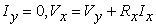and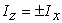where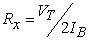and is, therefore, electronically-controllable through the external dc bias current IB.

The basic state-variable methodology to synthesize sinusoidal oscillators providing independent controls of FO and CO, as proposed in 5, can be reiterated as follows:

A canonic second-order (i.e. employing only two capacitors) oscillator can be characterized by the following autonomous state equation: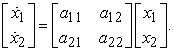(1)

From the above, the characteristic equation (CE)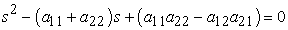(2)

gives the CO and FO as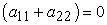(3)

and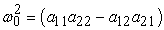(4)

The state variable synthesis methodology 5 involves, an a priori selection of the parameters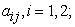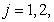in accordance with the required features (e.g. independent control for CO and FO through separate resistors), conversion of the resulting state equations into the node equations (NE) and finally, constructing a physical circuit from these NEs by using the chosen active building block and RC elements.

In the following, we choose the same types of matrices as introduced in 5, 6, 7, but employ the intrinsic input resistances Rx of the CCCIIs instead of the external resistors and demonstrate how a class of new electronically-controlled oscillators, not known earlier, can be systematically synthesized using this approach.

It must be kept in mind that in all the circuits derived in the following, the resistors Rx1, Rx2 and Rx3 are not the physical resistors but the intrinsic X-port input resistances of the CCCIIs employed which are electronically-controllable by respective external dc bias currents.

In the following, we first show the synthesis of one exemplary circuit explicitly and subsequently, the remaining types of [A] matrices suitable for the present purpose, along with the synthesized CCCII-based oscillators resulting therefrom and their COs and FOs would be presented directly in tabular form to conserve space.

For the realization of a current-controlled oscillator providing independent controls of both CO and FO, and following the ideas contained in 5, 6, 7, let us choose the elements of the [A] matrix as: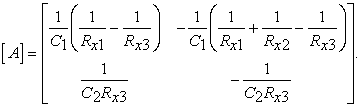(5)

From Eqn. 5, the characteristic equation of the oscillator, having [A] matrix as above, is given by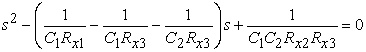(6)

from where the CO and FO are found to be: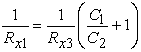(7)

and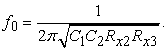(8)

Thus, as intended, indeed, CO and FO both are independently controllable without affecting each other; the former by Rx1 and the latter by Rx2.

Now, substituting eqn. (5) in (1), the resulting NEs can be written as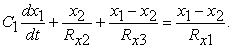(9)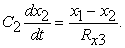(10)

By using NEs (9) and (10), a physical oscillator circuit is synthesized as shown in Figure 1, wherein the construction of the circuit can be understood by observing the various currents marked on the diagram itself.

• Figure 1. A new oscillator circuit realized by using NEs (9) and (10)

Since the CO is controllable by Rx1 while FO is independently controlled by Rx2, it follows that CO is electronically-controllable by IB1 whereas the FO is also independently electronically-controllable by IB2.

In Table 1 we now show the fourteen possible choices of the matrices [A] which lead to independent controls of both CO and FO. These matrices have been constructed on the basis of those given in 5, 6, 7 but here we have used the intrinsic resistances Rxi; i=1-3 of the CCCIIs rather than any external resistors. The Table 1 also shows the CO and FO of the synthesized oscillators which result from these matrices using the methodology as explained in the above example.

We can now write the following node equations resulting from the chosen 14 matrices of Table 1.

The NEs from [A] matrix 1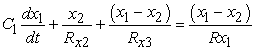(11a)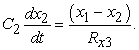(11b)

The NEs from [A] matrix 2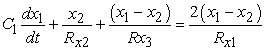(12a)(12b)

The NEs from [A] matrix 3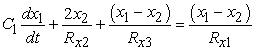(13a)(13b)

The NEs from [A] matrix 4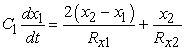(14a)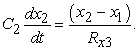(14b)

The NEs from [A] matrix 5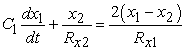(15a)(15b)

The NEs from [A] matrix 6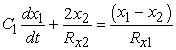(16a)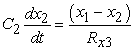(16b)

The NEs from [A] matrix 7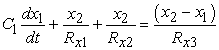(17a)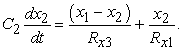(17b)

The NEs from [A] matrix 8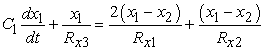(18a)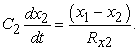(18b)

The NEs from [A] matrix 9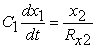(19a)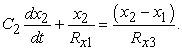(19b)

The NEs from [A] matrix 10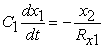(20a)(20b)

The NEs from [A] matrix 11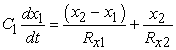(21a)(21b)

The NEs from [A] matrix 12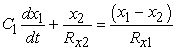(22a)(22b)

The NEs from [A] matrix 13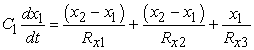(23a)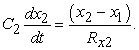(23b)

The NEs from [A] matrix 14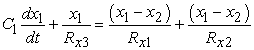(24a)(24b)

The oscillators synthesized from the above 14 sets of node equations are shown in Figure 2Figure 5. The CO and FO would be as already given in Table 1.

• Figure 2. New oscillator circuits realized by using NEs (11) to (14)
• Figure 3. New oscillator circuits realized by using NEs (15) to (18)
• Figure 4. New oscillator circuits synthesized from the NEs (19) to (22)
• Figure 5. New oscillator circuits synthesized from the NEs (23) and (24)

It may be mentioned that although three-CCCII-based oscillators possessing the intended properties mentioned in the Introduction, have been reported earlier also in references 24, 25, 27, 37 none of the fourteen topologies presented here have been known earlier and hence, are completely new.

### 3. Frequency Stability and Sensitivities

Frequency stability is an important figure of merit on the basis of which different sinusoidal oscillators can be compared. We use the definition of frequency stability factor as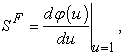where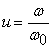is the normalized frequency and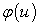represents the phase of the open loop transfer function of the oscillator circuit.

Here we show the derivation of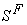for the circuit of Figure 1. The open loop transfer function (with the link broken at point ‘P’ as shown in Figure 1) is found to be: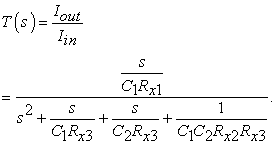(25)

Thus, from Eqn. (25), the phase of the T(s) with the selection of component values as C1=C2=C, 2Rx1=Rx3=Rx and Rx2=Rx/n, is given by: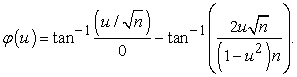(26)

Now, differentiating Eqn. (26) with respect to u, we get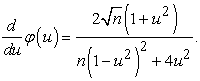(27)

From (27) the value of SF for this particular oscillator with u=1, is obtained to be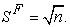(28)

Thus, the current-controlled oscillator of Figure 1 offers very good frequency stability factor for large values of n. By a similar analysis, it is found that the magnitude ofis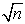for oscillator 7 also whereas for all the remaining oscillators,is found to be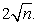Thus, all the new oscillators enjoy excellent frequency stability properties.

On the other hand, the sensitivity of ω0 with respect to Rxi; i=1-3 and the capacitances are found to be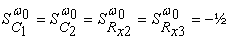and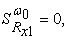which shows that all the oscillator circuits of Figure 2 to Figure 5 also enjoy very low sensitivity properties.

### 4. SPICE Simulation Results

To check the workability of the synthesized new current-controlled oscillator circuits, SPICE simulations have been performed using the bipolar transistor parameters of PR100N (PNP) and NR100N (NPN) transistors 41. All the circuits realized using MO-CCCII have been simulated in SPICE using the structure of the MO-CCCII shown in Figure 6, which is obtained by suitably augmenting the architecture proposed by Yuce, Kircay and Toker in 42. The capacitor values used were C1=C2=100nF and the CO was adjusted through the variation of Rx1 which is a function of the bias current IB1. The FO was varied through the change of Rx2 which is a function of the bias current IB2 and the value of Rx3 was fixed through IB3. The three MO-CCCIIs were biased with ±2.5V DC power supplies.

• Figure 6. MO-CCCII internal structure obtained by suitable augmentation of the circuit of 
• Figure 7. The variation of oscillation frequency with respect to bias current for the oscillator circuit of Figure 5(b) (a) Transient response (b) Steady state response (C1=C2=100pF, IB1=2.086µA, IB2=12.83µA and IB3=1.3µA) and (c) variation of FO w.r.t. IB2

The SPICE generated transient and steady state waveforms and the variation of FO w.r.t. IB2 were studied for all the fourteen oscillator circuits. With the exception of the circuit of Figure 4a which was found to be in latch-up{1}, all other circuits behaved as predicted by the theory.

To conserve space, we show here the simulation results only for the oscillator circuit of Figure 5(b). The output waveforms are shown in Figure 7(a) and Figure 7(b) whereas the variation of oscillation frequency w.r.t. IB2 is shown in Figure 7(c) by varying IB2 from 0.513µA to 115.47µA corresponding to which f0 was found to vary from 99.965 kHz to 1500.00 kHz. The circuit exhibited excellent correspondence between IB2 and f0. The % total harmonic distortion (THD) in the generated waveform, at the frequency of 499.95 kHz, was found to be 3.55%. The workability of the oscillator circuit of Figure 5(b) is, thus, established by these simulation results.

### 5. Comparison with Earlier Known CCCII-based Oscillators

A comparison of the generated new oscillator circuits with those previously reported in 23, 24, 25, 26, 27, 28, 29, 30, 31, 32, 33, 34, 35, 36, 37, 38, 39 is shown in Table 2 from where it is revealed that oscillators of 23, 29 suffer from the drawback of employing dissimilar type of active elements; those in 34, 36, 39 use more than three active elements; those in 28, 30, 31, 32, 35, 38 employ less than three CCCIIs but do not possess independent controls of both CO and FO. It turns out that only the earlier circuits of 24, 25, 27, 37 use only three CCCIIs and two grounded capacitors, however, none of the 14 oscillators presented here are found to exist in any of these earlier works 24, 25, 27, 37 and hence, are completely new.

From the data given in seventh column of Table 2, it is revealed that the new circuits appear to have an edge over the quoted ones in terms of their comparatively larger frequency range of operation 100 kHz-1.5 MHz.

Table 3 shows the operating frequency ranges and the % THD for all the fourteen synthesized oscillator circuits. From the data of all the 14 new circuits, it is found that comparatively, the circuit of Figure 5(b) appears to be the best in terms of largest possible tuning range, low THD and high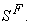It may be mentioned that like the three CCCII-based oscillators of 37 which are realised by using a CMOS CCCII architecture implementable in 0.35µm CMOS technology, the circuits of this paper can also be implemented with any chosen CMOS CCCII structure since the kernel of the work reported here is not dependent on the technology used.

Thus, the CCCII-based new oscillator circuits synthesized and reported in this paper compare well with the CCCII-based oscillators previously reported in 23, 24, 25, 26, 27, 28, 29, 30, 31, 32, 33, 34, 35, 36, 37, 38, 39, 40.

### 6. Concluding Remarks

Fourteen new electronically-controllable sinusoidal oscillator circuits have been synthesized by using the state-variable approach of 5, 6, 7. All the fourteen circuits have employed only three MO-CCCIIs and two grounded capacitors. The derived oscillator circuits provide the following desirable properties simultaneously: (i) employment of similar types of building blocks (ii) use of only three active elements (iii) availability of independent electronic controls of the FO and CO both (iv) complete elimination of external passive resistors and (v) the use of both grounded capacitor, as preferred for IC implementation. The workability of the proposed circuits has been verified by PSPICE simulations and some sample simulation results were presented. This paper has, thus, added 14 new oscillator structures to the existing repertoire of three CCCII-based electronically-controllable oscillators of 24, 25, 27, 37.

### Acknowledgements

This paper is based upon the work carried out partly at the Analog Signal Processing (ASP) Research Lab. of NSUT and partly at ASP Research Lab. of IET, Lucknow during 2016-2017.

### Note

1. The study of latch-up behavior in CCCII-based oscillators is, as yet, unstudied phenomenon and constitutes an interesting problem for further research.

### References

Published with license by Science and Education Publishing, Copyright © 2020 Manoj Kumar Jain, V. K. Singh and Raj SenaniThis work is licensed under a Creative Commons Attribution 4.0 International License. To view a copy of this license, visit http://creativecommons.org/licenses/by/4.0/